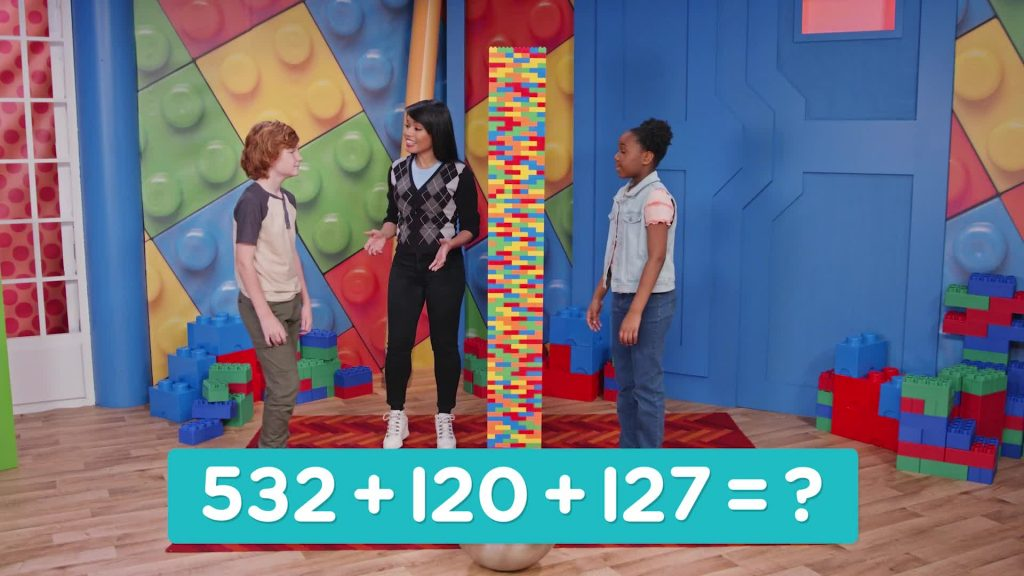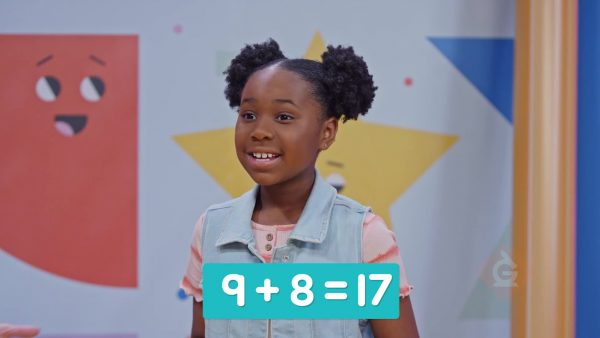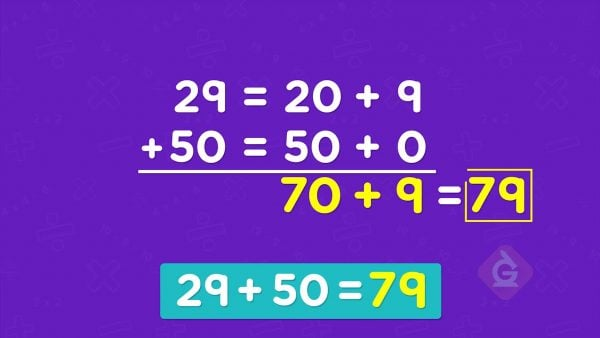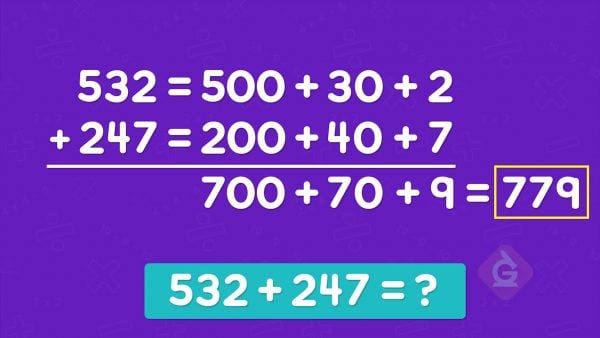Add More Than 2 Numbers | Math for Grades K, 1, & 2
1%
It was processed successfully!WHAT IS ADDING MORE THAN 2 NUMBERS?

You have already added 2 numbers together. Adding 3 or 4 numbers together is not much different at all!

To better understand adding more than 2 numbers…

WHAT IS ADDING MORE THAN 2 NUMBERS?. You have already added 2 numbers together. Adding 3 or 4 numbers together is not much different at all! To better understand adding more than 2 numbers…

## LET’S BREAK IT DOWN!To add 5 + 4 + 8, first add the first two numbers together. 5 + 4 = 9. Then add in the third number, 8. 9 + 8 = 17.

Add 3 numbers together. To add 5 + 4 + 8, first add the first two numbers together. 5 + 4 = 9. Then add in the third number, 8. 9 + 8 = 17.

### Add in a different order.You can add those same numbers in a different order. 4 + 8 = 12. Then add the 5. 5 + 12 = 17. The answer is the same.

Add in a different order. You can add those same numbers in a different order. 4 + 8 = 12. Then add the 5. 5 + 12 = 17. The answer is the same.

### You can break apart numbers to add.To add 11 + 18 + 50, break the numbers into tens and ones. 11 = 10 + 1 and 18 = 10 + 8.
Add the tens, then add the ones. 10 + 10 = 20. 1 + 8 = 9. The sum of the first two numbers is 20 + 9 = 29. Then add the 50. 29 + 50 = 79.

You can break apart numbers to add. To add 11 + 18 + 50, break the numbers into tens and ones. 11 = 10 + 1 and 18 = 10 + 8. Add the tens, then add the ones. 10 + 10 = 20. 1 + 8 = 9. The sum of the first two numbers is 20 + 9 = 29. Then add the 50. 29 + 50 = 79.

### To add 3-digit numbers, you can break numbers apart into hundreds, tens, and ones.Add 532 + 120 + 127. 532 = 500 + 30 + 2. 120 = 100 + 20. 127 = 100 + 20 + 7. Add the hundreds, tens, and ones separately, and then combine the place values. The sum is 700 + 70 + 9 = 779.

To add 3-digit numbers, you can break numbers apart into hundreds, tens, and ones. Add 532 + 120 + 127. 532 = 500 + 30 + 2. 120 = 100 + 20. 127 = 100 + 20 + 7. Add the hundreds, tens, and ones separately, and then combine the place values. The sum is 700 + 70 + 9 = 779.

## ADD MORE THAN 2 NUMBERS VOCABULARY

Place Value
The value of a digit based on its position in a number.
The process of putting parts together to find a new total.
Sum
Breaking Apart Numbers
The process of taking a number and writing it as the sum of 2 or more parts. You could break apart a number by place value (ex. 43 = 40 + 3) or break apart a number to help you make a ten (ex. 12 = 8 + 4).
Make a Ten
To choose and add numbers that make a tens number, like 10, 20, or 30.

## ADD MORE THAN 2 NUMBERS DISCUSSION QUESTIONS

### Add 6 + 5 + 7. What numbers did you add first?

Add 6 + 5 = 11. Then, add the third number. 11 + 7 = 18. The sum is 18.

### Add 15 + 47 + 5. What two numbers could you add first to make the problem easier to solve?

Add 15 + 5 first. 15 + 5 = 20. Then, add the other number. 20 + 47 = 67. The sum is 67.

### Add 13 + 19 + 7 + 21. What two pairs of numbers could you add first to make the problem easier to solve?

First, add 13 + 7 and 19 + 21. I know that 13 + 7 = 20. Add 19 + 21 = 19 + 1 + 20 = 20 + 20 = 40. Then, add 20 + 40 = 60. The sum is 60.

### Add 14 + 13 + 21 + 10.

I can start with any two numbers. I start with 14 + 13 = 27. Then, add in the next number. 27 + 21 = 48. Then, add the last number. 48 + 10 = 58.